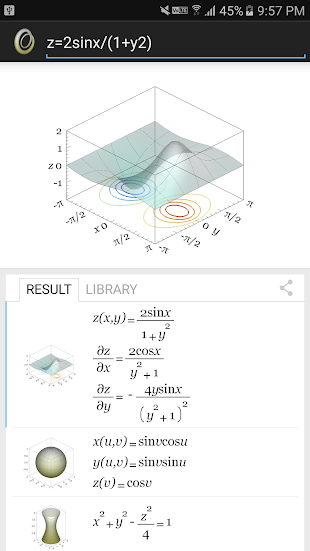# Newton Graphing Calculator Pro

9.98 usd

Why this app?We believe that calculating or drawing graph should be as intuitive as handwriting, without overhead in user input and complex syntax. For example, normally we write "sin2x" on paper, why we have to input "sin(2*x)" in a calculator. Wouldn't it be wonderful to just input "sin2x" in a calculator?Newton Graphing Calculator draws 2D curve and 3D surface by math input like handwriting. With our innovative parser, math syntax is as simple as when you write it on your note. 2D or 3D graph is recognized automatically by number of unknown variables, through our powerful symbolic math engine. After plotting, you can find function root, track coordinates, pan or zoom 2D graph; draw contour, zoom or rotate 3D graph to view from all angles seamlessly by hand gesture.
Newton Graphing Calculator comes with custom keyboard, high quality axis label, function legend, color picker and formatted math formula ready for productive use. Resulting graph and math formula can be sent by email to put into homework, shared with friend on Facebook, Line or Google+.
Newton Graphing Calculator features numerical calculator with step by step solution and live preview while inputting. Last session input is restored upon startup.
- Require media access permission, to store captured graph for sharing- Does not require internet access ― using embedded math engine
2D GRAPH• Plot explicit function e.g. y=sin2x+2cosx• Plot implicit function e.g. sin(x2+y)=exp(-xy)• Plot parametric function e.g. x=sin2t/(1+t2)   y=cos2t/(1+t2)• Plot polar function e.g. r=1+2cosθ• Plot multiple functions e.g. f=3sint/(1+t2)   g=2cost/(1+t2)• Plot multiple parametric function• Support discontinuity e.g. y=tanx• Variable name can be any symbol including Greek symbol
3D GRAPH• Plot explicit function e.g. z=2sinx/(1+y2)• Plot implicit function e.g. x2+y2+z2=1• Plot contour curve of explicit function e.g. z=2sinx/(1+y2)• Plot parametric function e.g. x=sinv cosu   y=sinv sinu   z=cosv• Plot multiple functions e.g. f=cosu sinv   g=ucosv• Plot multiple parametric function• Plot 3D curve x(t)   y(t)   z(t)• Variable name can be any symbol including Greek symbol
SCIENTIFIC CALCULATOR• Algebra, complex number, polynomial, rational function, 2D and 3D space, Newton-Raphson method• Scientific : pow sqrt root3 root4 abs exp log ln• Trigonometric : sin cos tan cot asin acos atan acot• Hyperbolic : sinh cosh tanh coth• Complex number e.g. (2i+1)(3i-1) ln(3i+4)• Derivative e.g. y=sin2x -> y' = 2cos2x